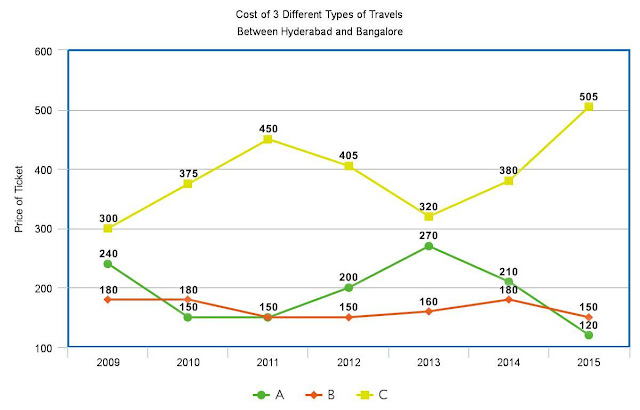New Students Offer - Use Code HELLO

# Data Interpretation Set For IBPS PO Preliminary 2016Click The Image To Zoom
1. In which of the following years the percentage increase/decrease in the cost of Travel C with respect to the previous year is the maximum?
1) 2012
2) 2015
3) 2010
4) 2011
5) None of these

2. In which of the following years the cost of all the three travels together is the maximum?
1) 2015
2) 2012
3) 2014
4) 2009
5) None of these

3. In which of the following two years the total cost of all three travels  is the same?
1) 2012 and 2015
2) 2012 and 2013
3) 2011 and 2013
4) 2010 and 2011
5) None of these

4. Find the percentage decrease in the cost of travel B from 2009 to 2015.
1) 20%
2) 15%
3) 24%
4) 16 %
5) None of these

5. Find the approximate difference between the average cost of travel A and that of travel B.
1) Rs. 23
2) Rs. 30
3) Rs. 33
4) Rs. 20
5) Rs. 27

1. 2
Maximum inclination shown between 2014 and 2015 hence answer is 2015

2. 1
Max in 2015 = 775

3. 3
1998 and 2000

4. 4
==> 30/180  * 10 0 = 50/3

5. 5
Req. difference  = (1340 - 1150)/7 = ~ 27